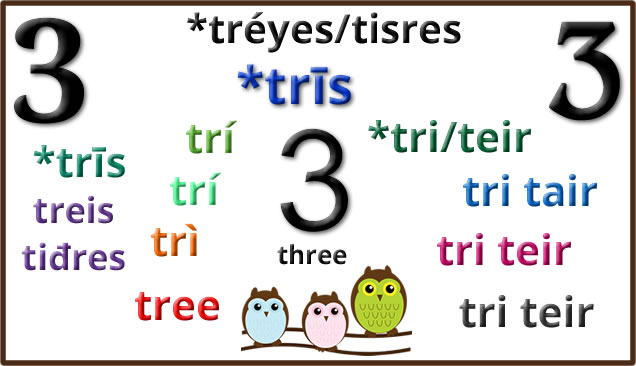# Threesome

Words for three and related things in Celtic languages:Proto-Celtic *trīs = three *tritiyos = third (in a sequence) *trisano- = third (fraction) Tiriś = three treis = three (m) tiđres = three (f) *trīs = three tri, trí [tʲrʲiː] = three tress = third (in a sequence) trían = third (fraction) tríar = three (people) tréide = three things trédenus = three days tréimse = three months trí = three tress = third (in a sequence), one of three trímad = third (in a sequence) trían = third (fraction) tríar = three persons, trio, three things tréimse = three months, a quarter (of a year) trí [tʲɾʲiː] = three tríú [tʲɾʲiːuː] = third (in a sequence), third part triúr [tʲɾʲuːɾˠ] = three (people) triantán = triangle triantánacht = trigonometry triantánaigh = to triangulate tríoiseach = three-dimensional trì [triː] = three treas [tres] (3ˢ) third (3ʳᵈ) treasamh (3ᵐʰ) third (3ʳᵈ) triùir [truːrʲ] = three (people) trì-fillte = triple, threefold, three-ply trian [triən] = third (part) triantan [triəndan] = triangle iantanachd [iantanachd] = trigonometry tree [t̪riː] = three troor = three (people), threesome, triad, Trinity, trio, trinity treeoo, trass = third trooane, troorane = triangle *tri [triː] = three (m) *teir = three (f) *trɨdɨð [trɨˈdɨːð] = third (m) *trɨdeð = third (f) *tri [triː] = three (m) *teir = three (f) tritid = third (in a sequence) trean = third (fraction) tri = three (m) teir, tair = three (f) trited, trydet, trydyd = third teir coglaỼc, trichonglaỼc, trichongloc = triangular, three-cornered trydeblyc, tridyblic = three times, threefold, triple tri [triː] = three (m), tair [tai̯r] = three (f) triawd = trio, threesome, triology, triple trichorn = three-horned, three-cornered, tricorn hat tric(h)onglog = triangular, three-cornered tri deg = thirty tridyblyg = three times, threefold, triple, triplicate trionglyn = triangle trydydd (3ydd) = third (m) trydedd (3edd) = third (f) teirgwaith = three times, thrice, on three occasions tri, trei = three (m) teir, teyr = three (f) triddydh = the space of three days tridzha = third trihans = three hundred trindas, trinsys = Trinity triugans = three score, sixty trivorh = three-pronged tri, trei = three (m) trei = three (f) tredhek, terdhek = thirteen tressa, tryja = third triasen = triplet trihorn = triangle tryflek = threefold, triple teyrgweyth = three times tri = three tri, try = three (m) teir, teyr = three (m) tri-c’hard = three quarters tri-ugent = sixty tri-chant = three hundred triad = trio, group of three tric’hogn, tricoign, tric’horn = triangle tri = three (m) teir [ˈte.iʁ] = three (f) trived (m) teirved (f) = third trizek = thirteen tri-ugent = sixty trifarzh = three quarters tric’horn, tric’hogn = triangle

Etymology: from Proto-Indo-European *tréyes (three – m) and *tisres (three – f) [source].

English words from the same roots include tertiary, three, thrice, three, triad, tripod, triple and triplex [source].

## 2 thoughts on “Threesome”

1.Robert says:

“English words from the same roots include tertiary, three, thrice, three, triad, tripod, triple and triplex”.

I see you noted “three” twice. At least it’s not thrice! Maybe that song idea about “twice” needs a second (or third!) look.

BTW, I seem to recall in my high school days (a tad and a smidgen over 50 years ago) that I read a book called “One, Two, Three, Infinity”. In its introduction, there was mention of some tribe that only had number words for one, two and three. Any amount over three was basically “many”. Are there still languages that are thusly so numerically challenged? I would be hesitant to label people with such a limited language as “primitive” but the word comes to mind.

Ever hear of such a thing?